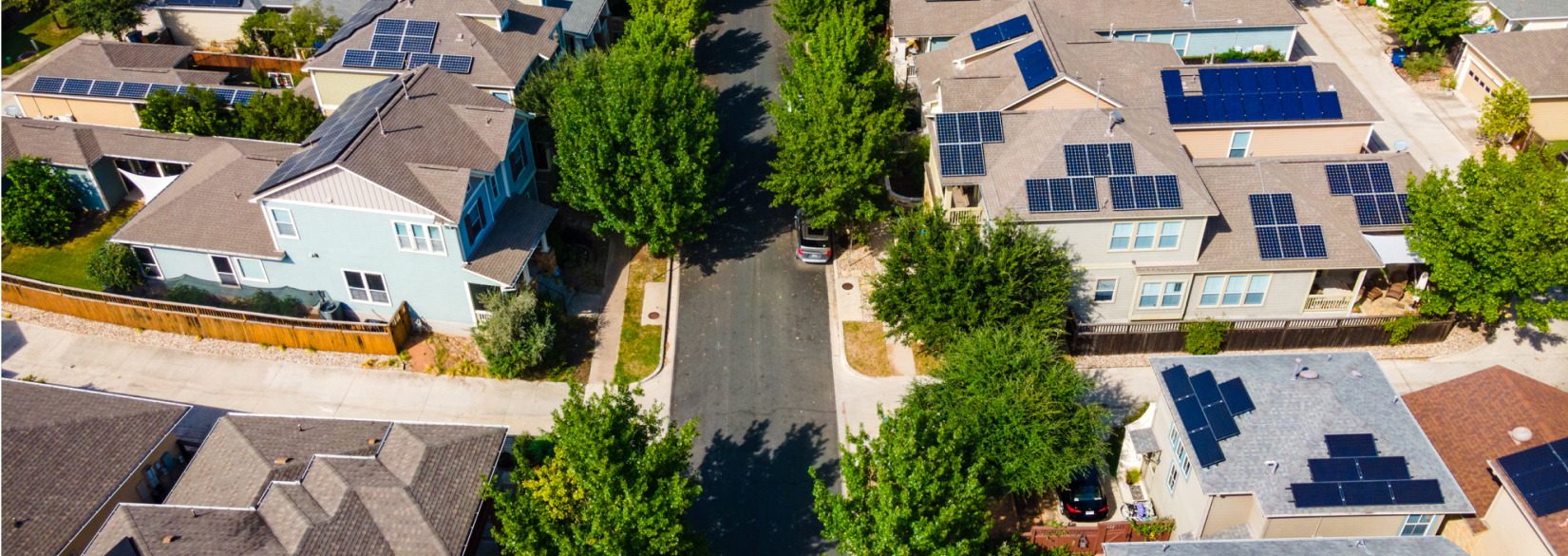# Case Study

## Houston Energy SystemsHouston Energy Systems is here to make your transition to solar as worry free as possible. We also take a different approach than traditional installers by …## Problem

Here is where you add the problems. Here is where you add the problems. Here is where you add the problems. Here is where you add the problems. Here is where you add the problems. Here is where you add the problems. Here is where you add the problems.## Solution

Here is where you add the problems. Here is where you add the problems. Here is where you add the problems. Here is where you add the problems. Here is where you add the problems. Here is where you add the problems. Here is where you add the problems.## Outcome

Here is where you add the problems. Here is where you add the problems. Here is where you add the problems. Here is where you add the problems. Here is where you add the problems. Here is where you add the problems. Here is where you add the problems.## Website Design and Development

You can add the contents for this project here.

### Logo

You can add the contents for this project here. You can add the contents for this project here. You can add the contents for this project here. You can add the contents for this project here. You can add the contents for this project here.